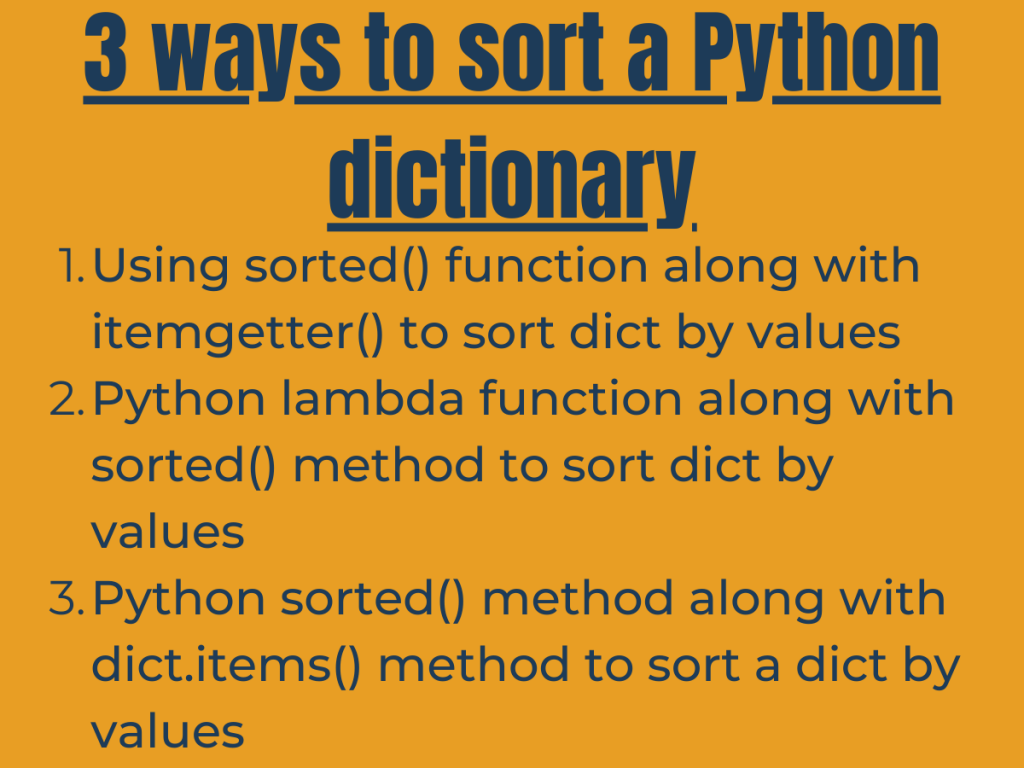# 3 Ways to Sort a Dictionary by Value in PythonHey, folks! In this article, we will be unveiling the steps to sort a dictionary by value in Python.

## Technique 1: Using sorted() function to sort a dictionary by value in Python

The `sorted() method` along with Python `operator.itemgetter() method` can be used to sort a dictionary by value in Python.

Python operator module has various in-built functions to manipulate the data and values. The operator.itemgetter(m) method considers the input object as an iterable and fetches all the ‘m’ values from the iterable.

Syntax: Python operator.itemgetter(m)

```operator.itemgetter(value)
```

Python sorted() method sorts the dict in an ascending/descending order.

Syntax: Python sorted() method

```sorted(iterable, key)
```
• `iterable`: It represents the elements to be sorted.
• `key`(optional): A function to decide the order of sorting the elements.

Example:

```from operator import itemgetter
inp_dict = { 'a':3,'ab':2,'abc':1,'abcd':0 }
print("Dictionary: ", inp_dict)
sort_dict= dict(sorted(inp_dict.items(), key=operator.itemgetter(1)))
print("Sorted Dictionary by value: ", sort_dict)
```

In the above function, we have set the parameter key = operator.itemgetter(1) because here ‘1’ represents the values of the dict, and ‘0’ represents the keys of the dict. Thus, we have sorted the dict by values in ascending order.

Output:

```Dictionary:  {'a': 3, 'ab': 2, 'abc': 1, 'abcd': 0}
Sorted Dictionary by value:  {'abcd': 0, 'abc': 1, 'ab': 2, 'a': 3}
```

## Technique 2: Python lambda function along with sorted() method

Python sorted() method can be clubbed along with lambda function to sort a dictionary by value in Python in a predefined order.

Python lambda function creates an anonymous function i.e. a function without a name. It helps optimize the code.

Syntax: Python lambda function

```lambda arguments: expression
```

Example:

```inp_dict = { 'a':3,'ab':2,'abc':1,'abcd':0 }
print("Dictionary: ", inp_dict)
sort_dict= dict(sorted(inp_dict.items(), key=lambda item: item))
print("Sorted Dictionary by value: ", sort_dict)
```

In the above snippet of code, we have created a lambda function and passed the values of the dict as argument to it by iterating through the dict by values i.e. item.

Output:

```Dictionary:  {'a': 3, 'ab': 2, 'abc': 1, 'abcd': 0}
Sorted Dictionary by value:  {'abcd': 0, 'abc': 1, 'ab': 2, 'a': 3}
```

## Technique 3: Python sorted() method along with dict.items()

Python sorted() function can be used to sort a dictionary by value in Python by passing the values through dict.items() to the method.

The `dict.items() method` fetches the keys/values from a dictionary.

Syntax:

```dict.items()
```

Example:

```inp_dict = { 'a':3,'ab':2,'abc':1,'abcd':0 }
print("Dictionary: ", inp_dict)
sort_dict= dict(sorted((value, key) for (key,value) in inp_dict.items()))
print("Sorted Dictionary by value: ", sort_dict)
```

In the above snippet of code, we have passed (value,key) pair to the sorted function and have fetched the dict values using dict.items() method. In this case, we would receive the sorted (value,key) pair as the output.

Output:

```Dictionary:  {'a': 3, 'ab': 2, 'abc': 1, 'abcd': 0}
Sorted Dictionary by value:  {0: 'abcd', 1: 'abc', 2: 'ab', 3: 'a'}
```

## Conclusion

Thus, in this article, we have understood the different ways to sort a dictionary by value in Python.

## References

• Python sort dict by values — JournalDev